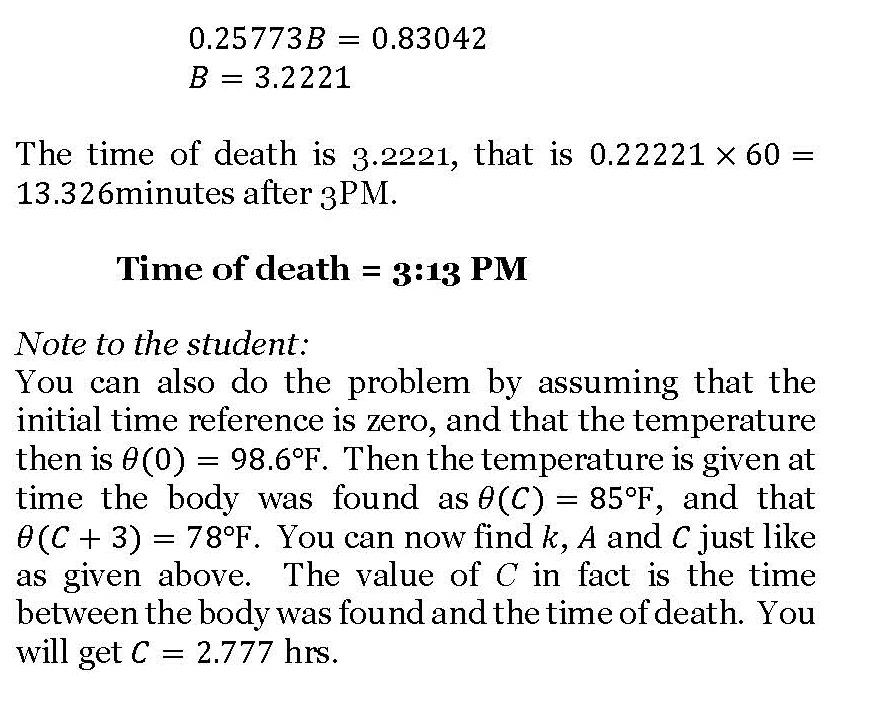# Time of death – a classic ODE problem

One of the classical applied problems in ordinary differential equations is that of finding the time of death of a homicide victim.

The estimation of time of death is generally based on the temperature of the body at two times – 1) when the victim is found and 2) then a few hours later. Assuming the ambient temperature stays the same and the body is treated as a lumped system, one can use a simple linear ODE to solve the problem.

It is somewhat an inverse problem as we are trying to find the value of the independent variable – the time of death. Here is a solved problem (a pdf version also available)._____________________________________________________

This post is brought to you by Holistic Numerical Methods: Numerical Methods for the STEM undergraduate at http://numericalmethods.eng.usf.edu

## Author: Autar Kaw

Autar Kaw (http://autarkaw.com) is a Professor of Mechanical Engineering at the University of South Florida. He has been at USF since 1987, the same year in which he received his Ph. D. in Engineering Mechanics from Clemson University. He is a recipient of the 2012 U.S. Professor of the Year Award. With major funding from NSF, he is the principal and managing contributor in developing the multiple award-winning online open courseware for an undergraduate course in Numerical Methods. The OpenCourseWare (nm.MathForCollege.com) annually receives 1,000,000+ page views, 1,000,000+ views of the YouTube audiovisual lectures, and 150,000+ page views at the NumericalMethodsGuy blog. His current research interests include engineering education research methods, adaptive learning, open courseware, massive open online courses, flipped classrooms, and learning strategies. He has written four textbooks and 80 refereed technical papers, and his opinion editorials have appeared in the St. Petersburg Times and Tampa Tribune.

## 4 thoughts on “Time of death – a classic ODE problem”

1. Denaya says:

Dear Sir,

According to what I’ve read on eqworld.ipmnet.ru site at http://eqworld.ipmnet.ru/forum/viewtopic.php?f=2&t=34&st=0&sk=t&amp;
on the topic of Mathematical Equations posted by Janesh, the linear first order DE of homicide victim model dӨ/dt + kӨ = kӨa corresponds to zero (n=0) order of Bernoulli DE. On her post, Janesh introduced a so-called Rohedi’s formula for Bernoulli DE, maybe the formula can be used to verify your exact solution. Let we read together the detail explanation from Janesh.

%%%%%%%%%%%%%%%%%%%%%%
Rohedi’s Formula For BDE of constant coefficients
Post by janesh on 03 Nov 2008, 21:27
Dear Sir,

I am so interested to Rohedi’s Formula for BDE of constant coefficients as you introduced in your SMT’s paper on http://rohedi.com/content/view/25/26/:

dy/dx + p*y = Q*y^n, for n is not equal 1

that is of form :

y(x) = [ {y0^(1-n) – Q/p}*exp{-(1-n)p(x-x0)} + Q/p ]^{1/(1-n)}

Can you give us some applications of the Rohedi’s formula?

Thanks Mr.Rohedi,
Janesh.
%%%%%%%%%%%%%%%%%%%%

Now, I try to verify your result using rohedi’s formula, where for the following DE

dӨ/dt + kӨ = kӨa , (1)

I have several parameters x=t, y=Ө, p=k, Q=kӨa, and n=0. Hence, the general solution of eq.(1) is in the form :

Ө(t) = {Ө0 – Q/p}*exp{-p(t-t0)} + Q/p

or

Ө(t) = {Ө0 – Өa}*exp{-p(t-t0)} + Өa , (2)

For k=0.25773, and Өa=72, then eq.(2) becomes

Ө(t) = ( Ө0 – 72)*exp{-0.25773(t-t0)} + 72 , (3)

Next, inserting your initial values t0=B, Ө0=98.6 into eq.(3), then the exact solution of homicide victim is of form:

Ө(t) = ( 98.6 – 72)*exp{-0.25773(t-3.2221)} + 72, (4)

Verify of eq(4) can be performed by inserting t=6 into eq(4), that gives Ө=85.

Okay Sir, your calculation is right, and I am very glad now.

Thanks Sir.

Astrid Denaya Lesa.

Like

2. Barbara says:

Thx Denaya Lesa.

Like

3. Denaya Lesa says:

Sorry, Denaya has just known that there is nice comment for me. But, I think Barbara also would thank to Professor Autar Kaw who has accepted me in posting Rohedi’s formula of my father here. Thx.